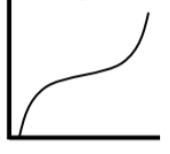### Home > GC > Chapter 1 > Lesson 1.1.2 > Problem1-16

1-16.

Match each table of data below with the most appropriate graph and briefly explain why it matches the data.

Time will always be shown on the $x\text{-axis}$.

1. Boiling water cooling down

 Time (min.) Temp. ($°$C) $0$ $5$ $10$ $15$ $20$ $25$ $100$ $89$ $80$ $72$ $65$ $59$

Determine if the $y\text{-values}$ are increasing or decreasing.

Since the numbers for the $y\text{-axis}$ are decreasing, the only graph which fits is Graph $3$.

2. Cost of a phone call

 Time (min.) Cost (cent.) $1$ $2$ $2.5$ $3$ $4$ $5$ $5.3$ $6$ $55$ $75$ $75$ $95$ $115$ $135$ $135$ $155$

Are the $y\text{-values}$ increasing at a constant rate?

This could be Graph $1$ or Graph $2$, but the table would not contain the point $(0,0)$, so it must be Graph $2$.

3. Growth of a baby in the womb

 Age (months) Length (inches) $1$ $2$ $3$ $4$ $5$ $6$ $7$ $8$ $9$ $0.75$ $1.5$ $3$ $6.4$ $9.6$ $12$ $13.6$ $15.2$ $16.8$

Identify the pattern of how the length increases.

Graph $4$ fits best.

Graph 1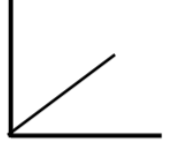Graph 2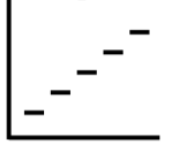Graph 3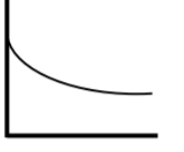Graph 4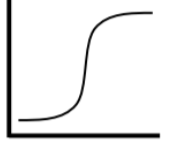Graph 5